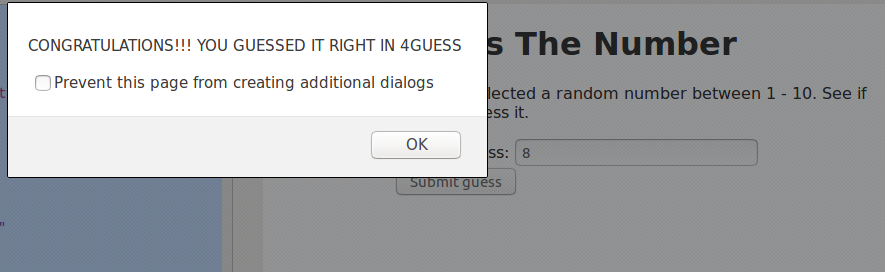# Number Guessing Game using JavaScript

• Difficulty Level : Easy
• Last Updated : 15 Feb, 2019

Prerequisites:

The game is to guess a random number generated by computer in range 1 – 10 in minimum number of Guesses.

Functions to be used:

1. document.getElementById(“id given”): document.getElementById() is used to fetch an element from the HTML page having the id as provided (specified) by the user.
“.value” is used to access the value of the HTML element accessed.

2. Math.random() : The random() function is used to generate a random number between 0 (inclusive) and 1 (exclusive). This generated number is then multiplied with 10 and added 1 to generate numbers from 1 – 10.

3. Math.floor() : The floor() function is used to return the number to the nearest integer (downwards). The value will not be rounded, if the passed argument is an integer.

Implementation of the Game.

 `                        ````````    ````    ``Number Guessing Game`` ` `    `````` ` ```

Guess The Number

`` ` `

We have selected a random number between 1 - 10. ``See ``if` `you can guess it.

`` ` `
``    ````    ````    ````
`` ` `````                    `

Output:My Personal Notes arrow_drop_up